Next: Bibliography Up: Derivation of Gauss planetary Previous: Perturbed orbit in standard

# Gauss planetary equations

At any given instance in time, a perturbed planetary orbit is completely determined by six osculating orbital elements. (See Section 10.2.) Let us choose these elements to be the major radius,; the mean anomaly at epoch,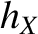; the eccentricity,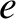; the argument of the perihelion,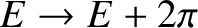; the inclination to the ecliptic,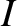; and the longitude of the ascending node,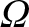. In the absence of a disturbing force,,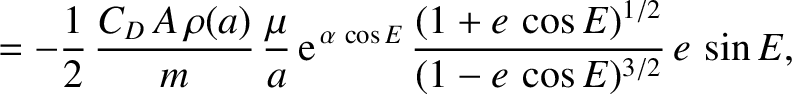,,,, andare all constants of the motion. As is clear from the analysis of the preceding two sections, in the presence of a disturbing force of the form (I.15), the osculating elements evolve in time as follows: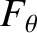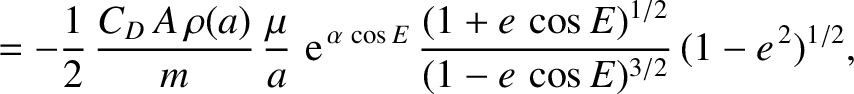(I.53)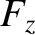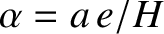(I.54)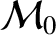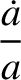(I.55)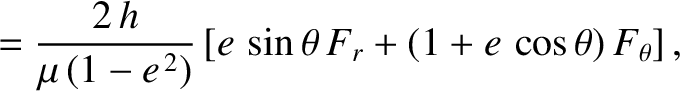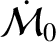(I.56)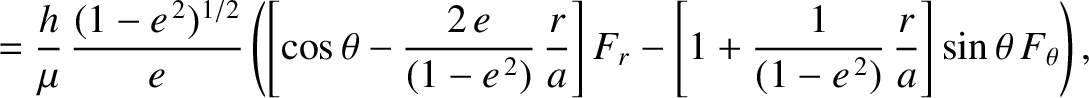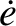(I.57) and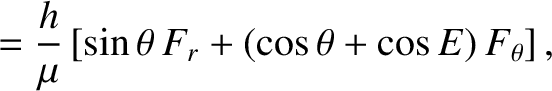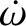(I.58)

These equations are known collectively as the Gauss planetary equations.Next: Bibliography Up: Derivation of Gauss planetary Previous: Perturbed orbit in standard
Richard Fitzpatrick 2016-03-31